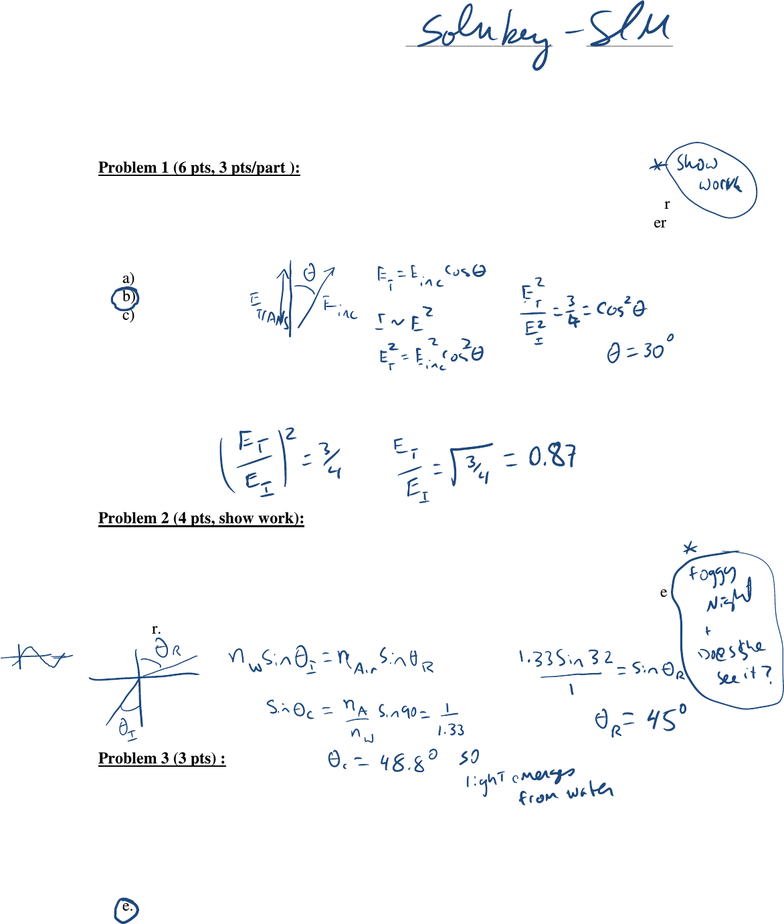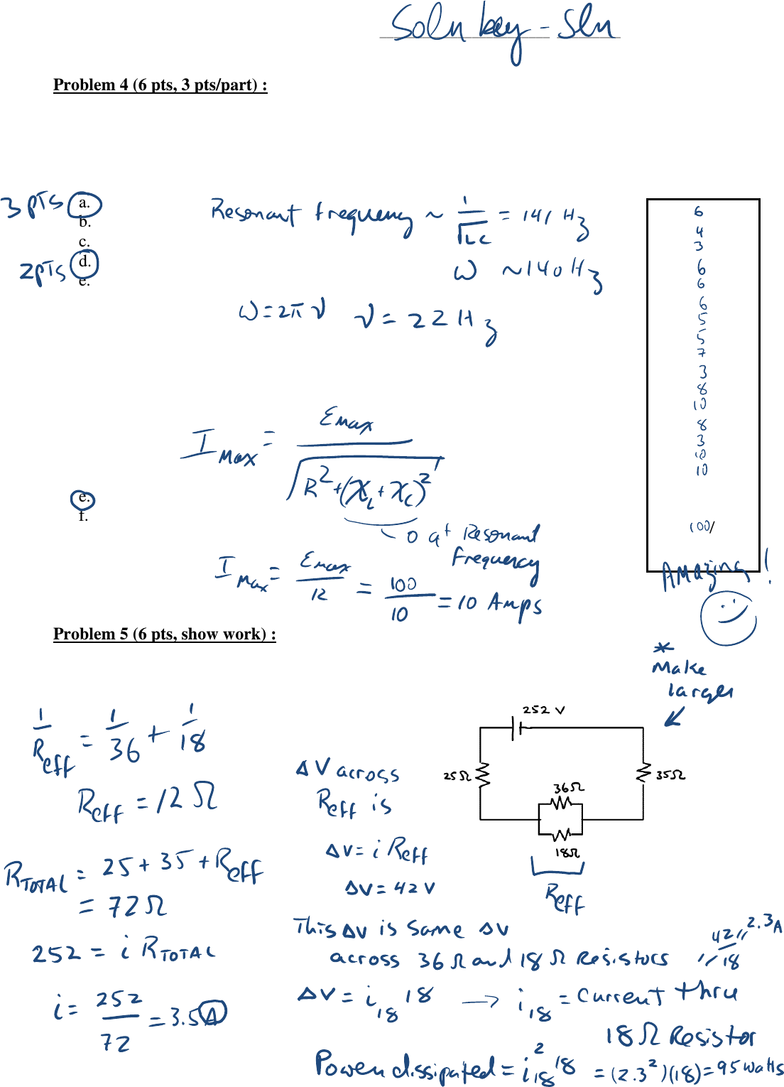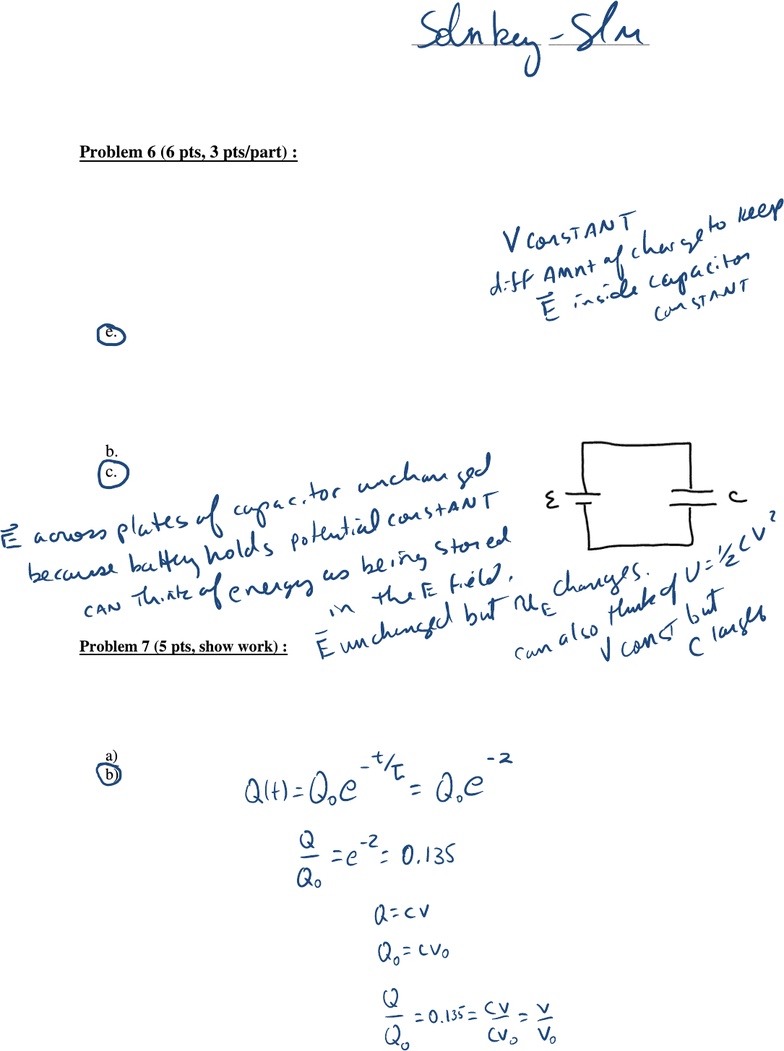Study Guides (400,000)
US (230,000)
U of R (600)
PHY (90)
All (8)
Final

# P142 FinalExam 2008 solnsExam

Department
Physics
Course Code
PHY 142
Professor
All
Study Guide
Final

This preview shows pages 1-2. to view the full 8 pages of the document.P142 University of Rochester NAME _________________________________________
S. Manly Fall 2008
Final Exam (December 19, 2008)
the back of the page, if necessary. Show all your work. Partial credit will be given.
Problem 1 (6 pts, 3 pts/part ):
Part I: Initially polarized light is incident on a polarizer. If the intensity of the light after
passing through the polarizer is ¾ of the initial intensity, the angle between the polarizer
axis and the original polarization axis of the light is approximately
a) 41.4 degrees
b) 30 degrees
c) 49 degrees
d) 60 degrees
Part II: What is the maximum electric field amplitude for the initial light relative to the
transmitted light?
Problem 2 (4 pts, show work):
Biff goes diving and shines an underwater searchlight at the surface of the water (index
of refraction 1.33) with an angle of incidence of 32 degrees relative to the normal to the
surface of the water. Buffy, sitting in a boat on the water, sees the light emerge from the
water making an angle of how many degrees with respect to the normal to the surface of
the water.
Problem 3 (3 pts) :
Ampere‟s law is valid
a. when there is a high degree of symmetry in the system.
b. when there is no symmetry in the system.
c. when the current is constant.
d. when the magnetic field is constant.
e. always.

Only pages 1-2 are available for preview. Some parts have been intentionally blurred.P142 University of Rochester NAME _________________________________________
S. Manly Fall 2008
Problem 4 (6 pts, 3 pts/part) :
A series RLC circuit with L=5 H, C=10 μF, and R =10 ohms is driven by a generator
with a maximum emf of 100V and a variable frequency. The resonant frequency of this
circuit is approximately
a. 22 Hz
b. 45 Hz
c. 90 Hz
d. 140 Hz
e. 180 Hz
f. 360 Hz
The maximum current in this circuit at resonance is approximately
a. 3.2 A
b. 5.3 A
c. 7.6 A
d. 9.5 A
e. 10 A
f. 12 A
Problem 5 (6 pts, show work) :
In the circuit to the right, what is the power dissipated in the 18 ohm resistor?
1) /6
2) /4
3) /3
4) /6
5) /6
6) /6
7) /5
8) /5
9) /7
10) /3
11) /8
12) /10
13) /8
14) /3
15) /10
16) /10
____________
tot /100

Unlock to view full version

Only pages 1-2 are available for preview. Some parts have been intentionally blurred.P142 University of Rochester NAME _________________________________________
S. Manly Fall 2008
Problem 6 (6 pts, 3 pts/part) :
A capacitor is connected to a battery as shown. When a dielectric is inserted between the
plates of the capacitor,
a. only the capacitance changes.
b. only the voltage across the capacitor changes.
c. only the charge on the capacitor changes
d. both the capacitance and the voltage change.
e. both the capacitance and the charge change.
The energy stored in the capacitor after the dielectric is inserted is ______ the energy
stored in the capacitor before the dielectric is inserted.
a. less than
b. equal to
c. greater than
Problem 7 (5 pts, show work) :
Sammy charges a capacitor and then discharges it through a resistor. He notices that, after at time
equal to two time constants passes, the voltage across the capacitor has decreased to roughly ____
of its value just prior to the initiation of the discharge.
a) 0.368
b) 0.135
c) 0.0498
d) 0.0183
e) 0.00674